## Areas of Triangles

The most common formula for finding the area of a triangle is K = ½ bh, where K is the area of the triangle, b is the base of the triangle, and h is the height. (The letter K is used for the area of the triangle to avoid confusion when using the letter A to name an angle of a triangle.) Three additional categories of area formulas are useful.

Two sides and the included angle (SAS): Given Δ ABC (Figure ), the height is given by h = c sinA. Therefore,Figure 1
Reference triangles for area formulas.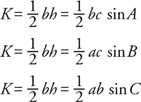Two angles and a side (AAS) or (ASA): Using the Law of Sines and substituting in the preceding three formulas leads to the following formulas: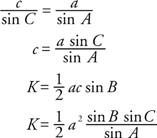Similarly,Three sides (SSS): A famous Greek philosopher and mathematician, Heron (or Hero), developed a formula that calculates the area of triangles given only the lengths of the three sides. This is known as Heron's formula. If a, b, and c are the lengths of the three sides of a triangle, and s is the semiperimeter, then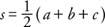and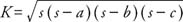One of many proofs of Heron's formula starts out with the Law of Cosines: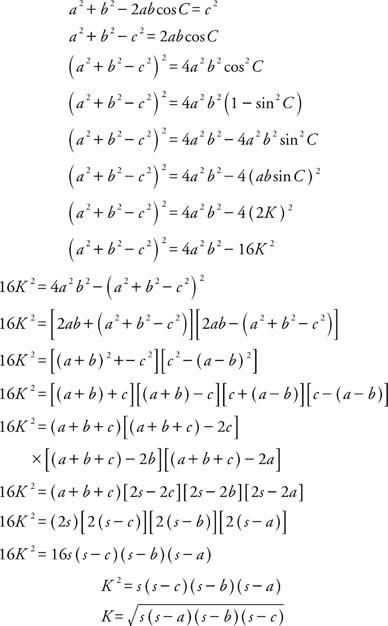Example 1: (SAS) As shown in Figure 2 , two sides of a triangle have measures of 25 and 12. The measure of the included angle is 51° Find the area of the triangle.Figure 2
Drawing for Example 1.

Use the SAS formula: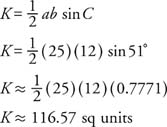Example 2: (AAS and ASA) Find the area of the triangle shown in FigureFigure 3
Drawing for Example 2.

First find the measure of the third angle of the triangle since all three angles are used in the area formula.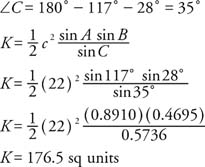Example 3: (AAS orASA) Find the area of an equilateral triangle with a perimeter of 78.

If the perimeter of an equilateral triangle is 78, then the measure of each side is 26. The nontrigonometric solution of this problem yields an answer of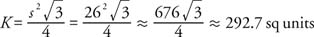The trigonometric solution yields the same answer.Example 4: (SSS) Find the area of a triangle if its sides measure 31, 44, and 60.

Use Heron's formula: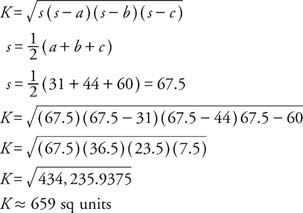Heron's formula does not use trigonometric functions directly, but trigonometric functions were used in the development and proof of the formula.